|

# 瞒不住了!这个能体验24小时日夜潮玩的家具展,你该解锁了!

白天很快乐，晚上很值得，奶茶当酒喝，聚众打火锅，家当游乐场......无论何时，这届年轻人就是要玩够24小时，果真很潮很疯很潇洒！

除此之外，年轻人的生活方式还能怎么玩？不如来3月最值得打卡的潮玩家具展了解一下！

3月18-21日，中国家博会（广州）X一兜糖家居APP联合主办的「年轻人的日与夜·广州」家居生活及设计潮玩展将在琶洲广交会展馆14.2号馆B11趣味开放！糖主邀请了立品设计创始人、设计总监郑铮作为潮玩展首席设计顾问，围绕潮玩创意理念，打造「日」、「夜」、「家」展览区，让你穿越日夜，感受城市生活与宅家生活的设计魅力。总想搞大事的糖主还召集了20+跨界潮牌、原创家居品牌及一众优秀的设计师，共同打造一场专属年轻人的狂欢嘉年华！

霓虹灯牌、怀旧海报、潮流集市、创意光影快闪......这些展会元素既彰显了老广文化的复古经典，也集合了年轻潮玩的现代风格。不仅超好逛，还能吃喝拍照打卡一条龙，给你一个新奇的24小时日夜体验。

话不多说，糖主这就抢先带你看！

一、还原城市生活 感受白日的年轻躁动

谈到年轻人的奇趣生活，那可是撑起了城市消费的半边天！逛街文化、奶茶咖啡成了小资生活的代名词，无数个穿越城市的网红店，网红小吃，折射出年轻人骨子里的“造反叛逆”，致力于寻找流行有趣的玩乐路线嗨到底！

所以在展会的「日」区里，年轻人的城市生活将被完美还原：喝奶茶、吃小食、逛集市、买好物....吃喝逛玩一条龙，各种潮流家居单品应有尽有。

茶饮小食吃不停 放松身心叹下茶

讲起“叹茶”还仅限于老广人的一盅两件？那你就OUT啦！年轻人手中那杯奶茶果饮表示不服，得为它单独设立「叹下茶」展示区，怒刷一波存在感。

在这里，不仅有新鲜好喝的奈雪的茶，它作为年轻人热爱的茶饮品牌之一，为逛展的你提供一杯好茶，一口软欧包！也能喝上一杯香浓味足的玩乐咖啡，在啡香四溢的氛围中欣赏老板挚爱的手办和玩具，何乐而不为？更有广州特色小吃元氏钵仔糕，突破了传统造型和口味，推出丰富口感和色彩鲜艳的糕点零嘴，边逛边吃可以消磨一整天！

说好的叹茶，当然还得有歇息休坐的书店一角。

糖主邀请广州联合书店入驻展区，这种人文、艺术、优质生活于一体的空间，能让你切身感受年轻文化对“叹茶”的重新诠释。

新潮玩意买不完 走走逛逛趁下墟

来到展会，一定不能错过「趁下墟」白日市集！老广经典的“趁墟”时光与年轻一族的“购物”生活碰撞融合成展区潮流集市，为“趁墟”一词灌输年轻涵义。

踏入市集区，你会看到超多好看又好买的家居设计品牌，钱包请拿好，小心秒掏空！想要颜值爆棚却实用无比的？这里有！只有你想不到，没有你看不到——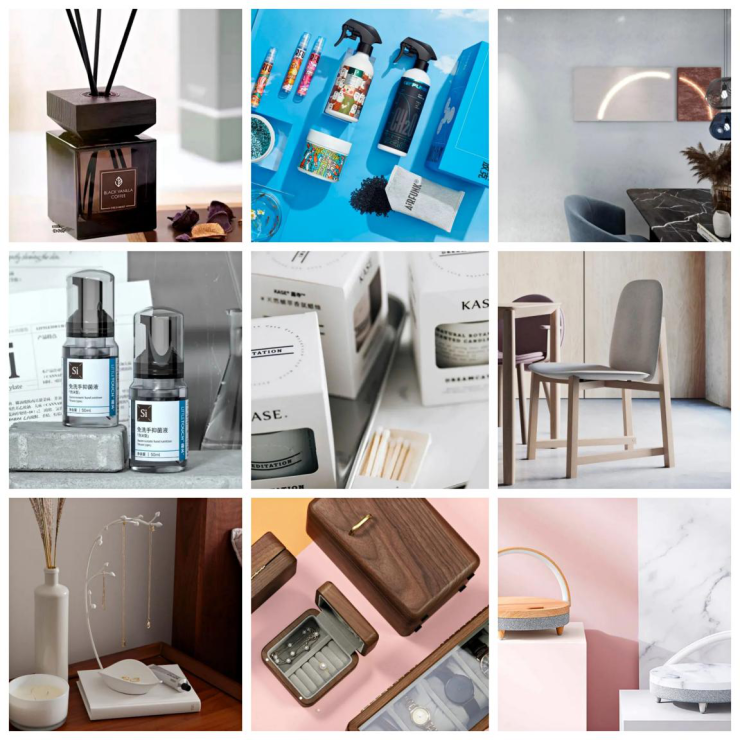打造美学功能兼备的薄荷部落，完全契合年轻人爱探寻、爱美的心态，打造清新舒适的颜值产品。强调愉悦呼吸体验的AIR FUNK，致力于改善居家空气环境问题，营造健康、放松的生活氛围。意大利原装进口涂料MP，不仅具备高超的涂料技术，还充满着独特的艺术情怀，展示出年轻人的多面形象。新锐国货品牌哩头，以丧萌可爱的漫画形象靠近年轻人，聚焦家居除菌、祛痘沐浴、光滑肌肤等问题，渗透年轻人的生活。注重艺术香氛的KASE嘉私，用香味协调年轻人的情绪，以更为生动、有趣的表现方式带来一种新的产品体验与生活方式。

想要设计极简且时尚流行的？这里也有！家居产品样样全，全都想抱回家——

简洁干净的Lepōs Design，将热情向上、淳朴温暖的设计特色融入每件家居产品中，倾注富含人性化的设计灵魂。休闲现代的Umbra，带来一系列做工精细、创意设计的家居产品，大大丰富了年轻人的家居空间。造型独特的创木工房，欣赏并关注着年轻人的喜好和行为细节，用创意想法设计出一个个令人惊叹且好玩的家居摆件。创意小电几光，凭借其对科技的创新以及家居美学的追求，打造了多款实力爆品，在年轻人群体中大受欢迎。高端垃圾桶优百纳（Upella），融入年轻人居家生活的“收纳”理念，与高端设计师们一同打造时尚简约的垃圾桶产品。

想要好玩新兴又无与伦比的？这里都有！每件风格各异，绝不容易撞款撞型——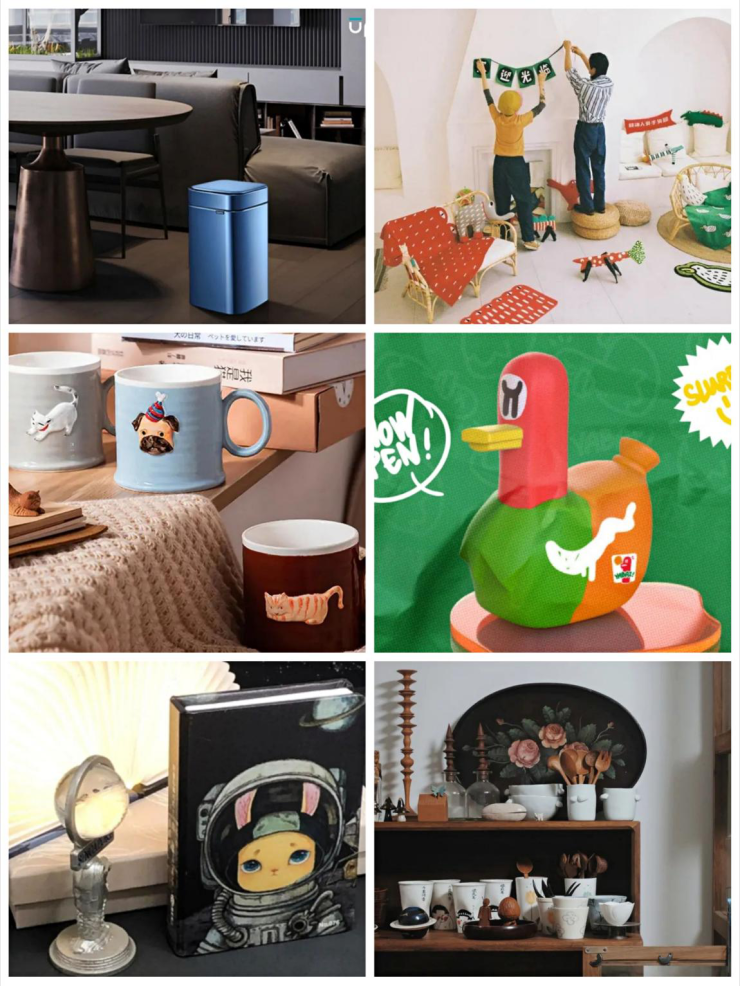独特有趣的林李婆婆，是广州青年艺术家林李的原创品牌，她创作出款式丰富的艺术衍生品，为单调、沉闷的生活增添一丝活泼的色彩。时尚精选平台简艾生活，精选年轻人最爱的潮流家居好物，号召他们用多元化的产品搭配家居空间，去表达自我，接纳自我，回归自我。知名玩具厂牌NIKU PARK，致力于创造一个充满可能性、天马行空、有趣的乐园，通过玩具载体发现年轻人的有趣生活。本土文创品牌PaperCare，在速朽时代让纸张的魅力永存，联合独艺无二出品的艺术家主题限量书灯2021年潮款首发，以文创载体传达纸张文化，配得上年轻人的青睐和善待。文艺气息浓郁的谷音茶饭事，用手工制成各式餐具，留下不少手工痕迹，这种人情味也时时向年轻人透露出好好吃饭的嘱咐。

创意未来家空间 体验入夜的宅家乐趣

不过对于年轻人来说，家才是最棒的游乐场。穿着睡衣在客厅健身，开着音乐，点上香氛再沉沉入睡。无论如何，他们的宅家空间早已突破传统：客厅不像客厅、极简卧室兴起、开放餐厨融合、收纳精细有序......原来在年轻人身上发生如此新颖有趣的宅家变化！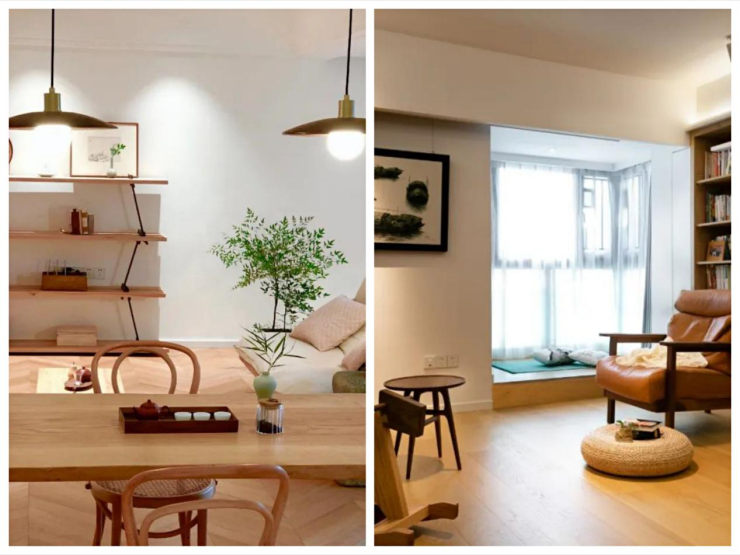而为了让大家逛展体验更深，年轻人对家的想象将会在展会的「夜」区里完成改造、创新和落地。

打造沉浸式场景 原来还能这样宅家

根据一兜糖家居APP发布的「家居生活及消费趋势报告」，多名设计师联手用创意脑洞打造3个沉浸式宅家场景，带你走进理想中的未来家。

色彩斑斓、变化无穷的「万花筒」景象经过设计师草三冉的创意还原，融合打造出年轻人疯玩无限、风格多变的客厅场景。深点设计创始人郑小馆则把客厅幻想成连接现实和梦境的盒子，表现出空间变化的对立、融合、温暖、神秘，释放出年轻人关于未来客厅的精神想象。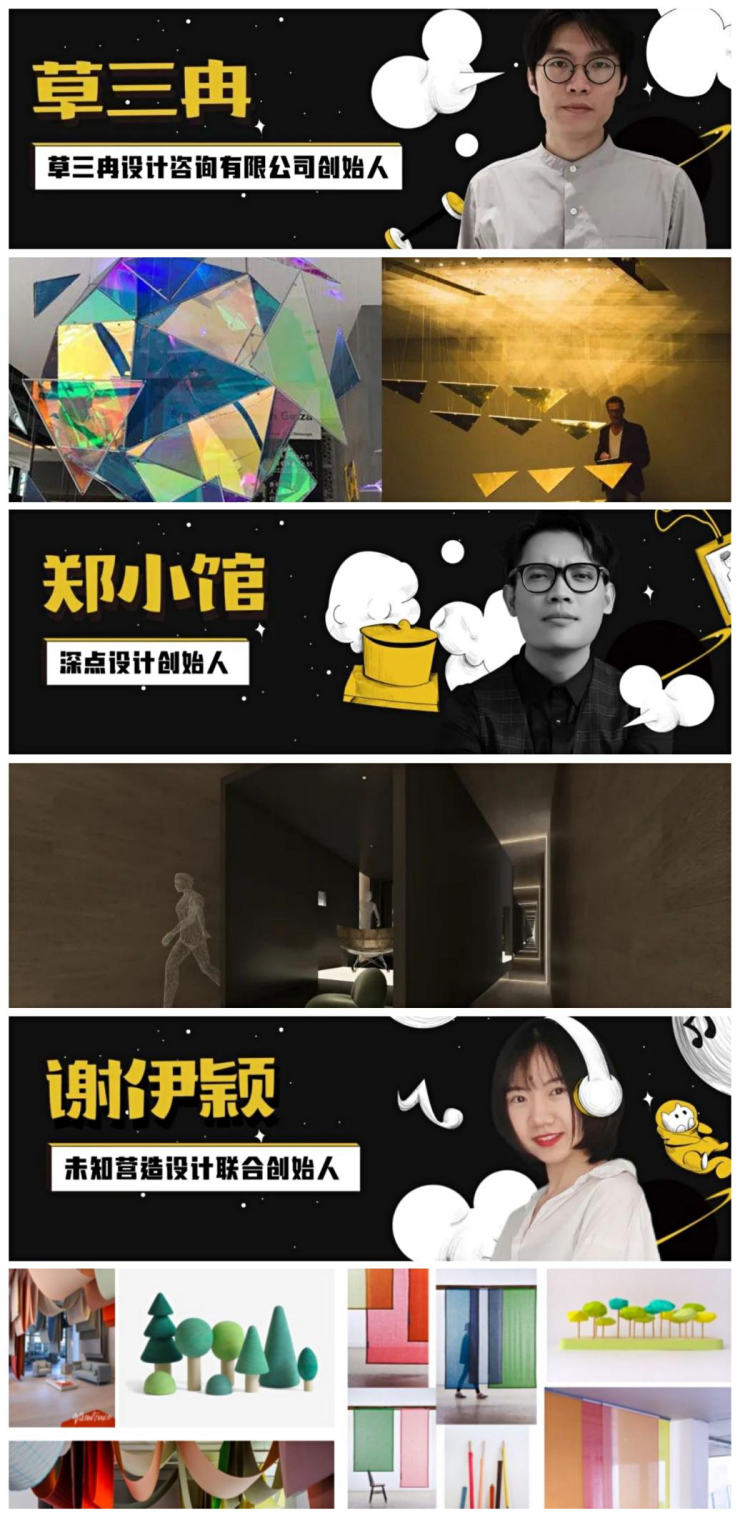为了体味睡眠时光的春夏交替，未知营造设计联合创始人谢伊颖用色彩展示轻松愉悦的入眠氛围，充分展示舒适卧室空间氛围，让来展的你感受午夜的浪漫。

而场景打造也绝对少不了这些家居品牌的身影——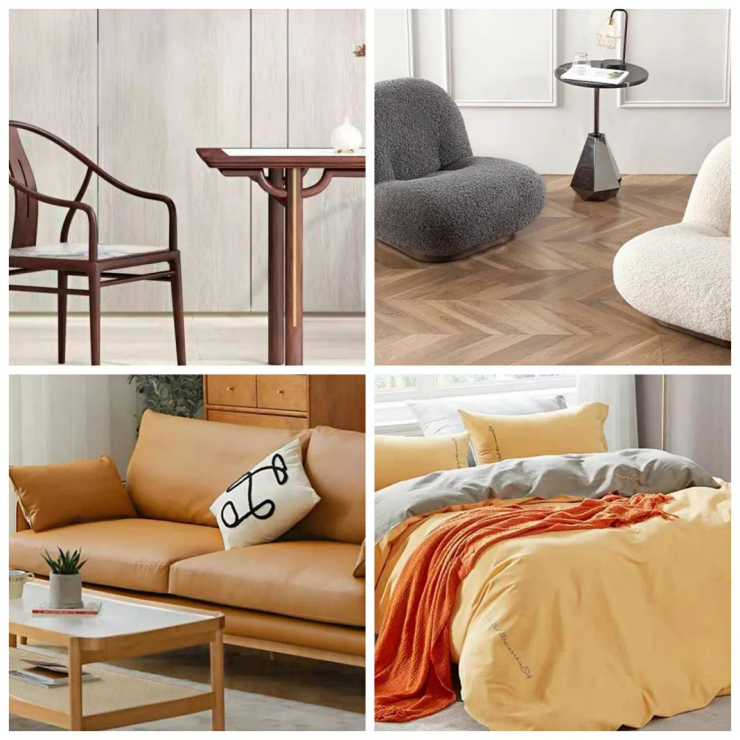联邦造，新国潮！联邦家私以新产品、新设计呈现新国际东方美学，让时尚生活 ，[潮]您而来！追求新意式美学的顾全家居，很好地匹配了新青年的生活方式，用有趣的消费塑造理想生活，也为传统欧式家居注入新鲜血液。日式实用派的北陌家具，坚持实木造家，专为生活习惯设计。品牌愿做生活新趋势的探路人，发现新习惯，质造新生活。以国粹诠释舒适睡眠的南方寝饰，致力于打造年轻人热爱的国潮设计家纺床品，追求品质睡眠生活。

炫酷灯展+互动打卡 随心挑战随心玩

另外，糖主还联合宝照灯饰、峰米科技一起打造创意无限的「睇下灯」展示区，玩出花样，玩出视觉享受，烘托出年轻人野性朝气的入夜生活。不得不说，宝照灯饰以新颖、会心、时尚的设计语言，通过挖掘年轻人夜生活的丰富性，让灯展的霓虹灯们显得五光十色、光怪陆离。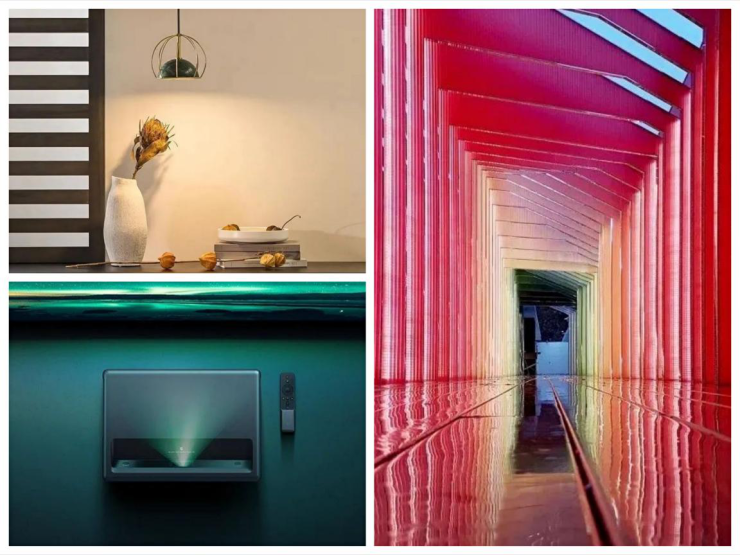而峰米科技则利用前沿的激光技术，构筑年轻人的未来生活想象，打造一幅充满科技的光影盛况。

来展会的你们想刷爆朋友圈，绝对要到灯光展区摆好Pose拍靓照，来场脑洞大开的“到此一游”。好玩不止于此，还有奖品多多的线上互动、线下打卡玩法等你来挑战！

凡是报名展会的糖友都可以凭展报名截图私信社群运营官糯米糖，领取专属领奖券，只需线下凭券去一兜糖摊位就能100%领奖，仅限200名，先到先得！

更有趣的是，来逛展的你们还可以人手一份展区地图，只要去往三大打卡区进行盖章，就可以到一兜糖摊位抽奖一次，礼物送不停！

除打卡三大区外，要是想100%拿奖，还能到指定5个参展品牌打卡盖章，拍照发朋友圈，完成这三大任务后，就可以到一兜糖摊位拿奖品了！*每天限100份奖品听起来是不是简单之余又好玩？快来报名逛展，千元奖品包你拿到手软。

赶紧约上三五好友去逛这个独一无二的「年轻人日与夜·广州」家居生活及设计潮玩展，糖主在摊位等你一起耍！

（文章来源：消费日报网）

`声明：本文由入驻焦点开放平台的作者撰写，除焦点官方账号外，观点仅代表作者本人，不代表焦点立场错误信息举报电话： 400-099-0099，邮箱：jubao@vip.sohu.com，或点此进行意见反馈，或点此进行举报投诉。`A B C D E F G H J K L M N P Q R S T W X Y Z
A - B - C - D - E
• A
• 鞍山
• 安庆
• 安阳
• 安顺
• 安康
• 澳门
• B
• 北京
• 保定
• 包头
• 巴彦淖尔
• 本溪
• 蚌埠
• 亳州
• 滨州
• 北海
• 百色
• 巴中
• 毕节
• 保山
• 宝鸡
• 白银
• 巴州
• C
• 承德
• 沧州
• 长治
• 赤峰
• 朝阳
• 长春
• 常州
• 滁州
• 池州
• 长沙
• 常德
• 郴州
• 潮州
• 崇左
• 重庆
• 成都
• 楚雄
• 昌都
• 慈溪
• 常熟
• D
• 大同
• 大连
• 丹东
• 大庆
• 东营
• 德州
• 东莞
• 德阳
• 达州
• 大理
• 德宏
• 定西
• 儋州
• 东平
• E
• 鄂尔多斯
• 鄂州
• 恩施
F - G - H - I - J
• F
• 抚顺
• 阜新
• 阜阳
• 福州
• 抚州
• 佛山
• 防城港
• G
• 赣州
• 广州
• 桂林
• 贵港
• 广元
• 广安
• 贵阳
• 固原
• H
• 邯郸
• 衡水
• 呼和浩特
• 呼伦贝尔
• 葫芦岛
• 哈尔滨
• 黑河
• 淮安
• 杭州
• 湖州
• 合肥
• 淮南
• 淮北
• 黄山
• 菏泽
• 鹤壁
• 黄石
• 黄冈
• 衡阳
• 怀化
• 惠州
• 河源
• 贺州
• 河池
• 海口
• 红河
• 汉中
• 海东
• 怀来
• I
• J
• 晋中
• 锦州
• 吉林
• 鸡西
• 佳木斯
• 嘉兴
• 金华
• 景德镇
• 九江
• 吉安
• 济南
• 济宁
• 焦作
• 荆门
• 荆州
• 江门
• 揭阳
• 金昌
• 酒泉
• 嘉峪关
K - L - M - N - P
• K
• 开封
• 昆明
• 昆山
• L
• 廊坊
• 临汾
• 辽阳
• 连云港
• 丽水
• 六安
• 龙岩
• 莱芜
• 临沂
• 聊城
• 洛阳
• 漯河
• 娄底
• 柳州
• 来宾
• 泸州
• 乐山
• 六盘水
• 丽江
• 临沧
• 拉萨
• 林芝
• 兰州
• 陇南
• M
• 牡丹江
• 马鞍山
• 茂名
• 梅州
• 绵阳
• 眉山
• N
• 南京
• 南通
• 宁波
• 南平
• 宁德
• 南昌
• 南阳
• 南宁
• 内江
• 南充
• P
• 盘锦
• 莆田
• 平顶山
• 濮阳
• 攀枝花
• 普洱
• 平凉
Q - R - S - T - W
• Q
• 秦皇岛
• 齐齐哈尔
• 衢州
• 泉州
• 青岛
• 清远
• 钦州
• 黔南
• 曲靖
• 庆阳
• R
• 日照
• 日喀则
• S
• 石家庄
• 沈阳
• 双鸭山
• 绥化
• 上海
• 苏州
• 宿迁
• 绍兴
• 宿州
• 三明
• 上饶
• 三门峡
• 商丘
• 十堰
• 随州
• 邵阳
• 韶关
• 深圳
• 汕头
• 汕尾
• 三亚
• 三沙
• 遂宁
• 山南
• 商洛
• 石嘴山
• T
• 天津
• 唐山
• 太原
• 通辽
• 铁岭
• 泰州
• 台州
• 铜陵
• 泰安
• 铜仁
• 铜川
• 天水
• 天门
• W
• 乌海
• 乌兰察布
• 无锡
• 温州
• 芜湖
• 潍坊
• 威海
• 武汉
• 梧州
• 渭南
• 武威
• 吴忠
• 乌鲁木齐
X - Y - Z
• X
• 邢台
• 徐州
• 宣城
• 厦门
• 新乡
• 许昌
• 信阳
• 襄阳
• 孝感
• 咸宁
• 湘潭
• 湘西
• 西双版纳
• 西安
• 咸阳
• 西宁
• 仙桃
• 西昌
• Y
• 运城
• 营口
• 盐城
• 扬州
• 鹰潭
• 宜春
• 烟台
• 宜昌
• 岳阳
• 益阳
• 永州
• 阳江
• 云浮
• 玉林
• 宜宾
• 雅安
• 玉溪
• 延安
• 榆林
• 银川
• Z
• 张家口
• 镇江
• 舟山
• 漳州
• 淄博
• 枣庄
• 郑州
• 周口
• 驻马店
• 株洲
• 张家界
• 珠海
• 湛江
• 肇庆
• 中山
• 自贡
• 资阳
• 遵义
• 昭通
• 张掖
• 中卫

1室1厅1厨1卫1阳台

1
2
3
4
5

0
1
2

1

1

0
1
2
3报名成功，资料已提交审核A B C D E F G H J K L M N P Q R S T W X Y Z
A - B - C - D - E
• A
• 鞍山
• 安庆
• 安阳
• 安顺
• 安康
• 澳门
• B
• 北京
• 保定
• 包头
• 巴彦淖尔
• 本溪
• 蚌埠
• 亳州
• 滨州
• 北海
• 百色
• 巴中
• 毕节
• 保山
• 宝鸡
• 白银
• 巴州
• C
• 承德
• 沧州
• 长治
• 赤峰
• 朝阳
• 长春
• 常州
• 滁州
• 池州
• 长沙
• 常德
• 郴州
• 潮州
• 崇左
• 重庆
• 成都
• 楚雄
• 昌都
• 慈溪
• 常熟
• D
• 大同
• 大连
• 丹东
• 大庆
• 东营
• 德州
• 东莞
• 德阳
• 达州
• 大理
• 德宏
• 定西
• 儋州
• 东平
• E
• 鄂尔多斯
• 鄂州
• 恩施
F - G - H - I - J
• F
• 抚顺
• 阜新
• 阜阳
• 福州
• 抚州
• 佛山
• 防城港
• G
• 赣州
• 广州
• 桂林
• 贵港
• 广元
• 广安
• 贵阳
• 固原
• H
• 邯郸
• 衡水
• 呼和浩特
• 呼伦贝尔
• 葫芦岛
• 哈尔滨
• 黑河
• 淮安
• 杭州
• 湖州
• 合肥
• 淮南
• 淮北
• 黄山
• 菏泽
• 鹤壁
• 黄石
• 黄冈
• 衡阳
• 怀化
• 惠州
• 河源
• 贺州
• 河池
• 海口
• 红河
• 汉中
• 海东
• 怀来
• I
• J
• 晋中
• 锦州
• 吉林
• 鸡西
• 佳木斯
• 嘉兴
• 金华
• 景德镇
• 九江
• 吉安
• 济南
• 济宁
• 焦作
• 荆门
• 荆州
• 江门
• 揭阳
• 金昌
• 酒泉
• 嘉峪关
K - L - M - N - P
• K
• 开封
• 昆明
• 昆山
• L
• 廊坊
• 临汾
• 辽阳
• 连云港
• 丽水
• 六安
• 龙岩
• 莱芜
• 临沂
• 聊城
• 洛阳
• 漯河
• 娄底
• 柳州
• 来宾
• 泸州
• 乐山
• 六盘水
• 丽江
• 临沧
• 拉萨
• 林芝
• 兰州
• 陇南
• M
• 牡丹江
• 马鞍山
• 茂名
• 梅州
• 绵阳
• 眉山
• N
• 南京
• 南通
• 宁波
• 南平
• 宁德
• 南昌
• 南阳
• 南宁
• 内江
• 南充
• P
• 盘锦
• 莆田
• 平顶山
• 濮阳
• 攀枝花
• 普洱
• 平凉
Q - R - S - T - W
• Q
• 秦皇岛
• 齐齐哈尔
• 衢州
• 泉州
• 青岛
• 清远
• 钦州
• 黔南
• 曲靖
• 庆阳
• R
• 日照
• 日喀则
• S
• 石家庄
• 沈阳
• 双鸭山
• 绥化
• 上海
• 苏州
• 宿迁
• 绍兴
• 宿州
• 三明
• 上饶
• 三门峡
• 商丘
• 十堰
• 随州
• 邵阳
• 韶关
• 深圳
• 汕头
• 汕尾
• 三亚
• 三沙
• 遂宁
• 山南
• 商洛
• 石嘴山
• T
• 天津
• 唐山
• 太原
• 通辽
• 铁岭
• 泰州
• 台州
• 铜陵
• 泰安
• 铜仁
• 铜川
• 天水
• 天门
• W
• 乌海
• 乌兰察布
• 无锡
• 温州
• 芜湖
• 潍坊
• 威海
• 武汉
• 梧州
• 渭南
• 武威
• 吴忠
• 乌鲁木齐
X - Y - Z
• X
• 邢台
• 徐州
• 宣城
• 厦门
• 新乡
• 许昌
• 信阳
• 襄阳
• 孝感
• 咸宁
• 湘潭
• 湘西
• 西双版纳
• 西安
• 咸阳
• 西宁
• 仙桃
• 西昌
• Y
• 运城
• 营口
• 盐城
• 扬州
• 鹰潭
• 宜春
• 烟台
• 宜昌
• 岳阳
• 益阳
• 永州
• 阳江
• 云浮
• 玉林
• 宜宾
• 雅安
• 玉溪
• 延安
• 榆林
• 银川
• Z
• 张家口
• 镇江
• 舟山
• 漳州
• 淄博
• 枣庄
• 郑州
• 周口
• 驻马店
• 株洲
• 张家界
• 珠海
• 湛江
• 肇庆
• 中山
• 自贡
• 资阳
• 遵义
• 昭通
• 张掖
• 中卫• 手机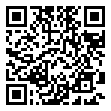• 分享
• 设计
免费设计
• 计算器
装修计算器
• 入驻
合作入驻
• 联系
联系我们
• 置顶
返回顶部